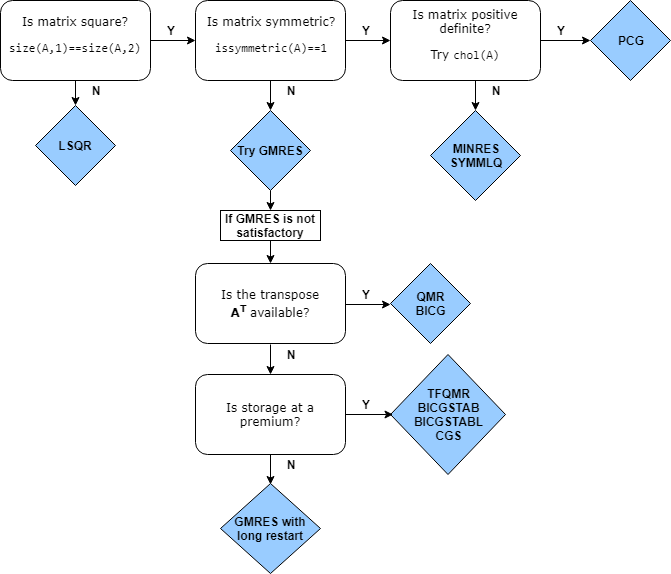## 线性方程组的迭代方法

### 直接方法与迭代方法

• 直接方法是高斯消去法的变体。这些方法通过矩阵运算（例如 LU、QR 或 Cholesky 分解）直接使用各个矩阵元素。您可以使用直接方法来求解具有高精度水平的线性方程，但在大型稀疏矩阵上运行这些方法时，速度可能会很慢。用直接方法求解线性方程组的速度很大程度上取决于系数矩阵的密度和填充模式。

例如，以下代码用于求解一个较小的线性方程组。

```A = magic(5); b = sum(A,2); x = A\b; norm(A*x-b)```
```ans = 1.4211e-14```

MATLAB 通过矩阵除法运算符 `/``\` 以及 `decomposition``lsqminnorm``linsolve` 等函数实现直接的方法。

• 迭代方法在经过有限的步数之后生成线性方程组的逼近解。这些方法对大型方程组非常有用，在求解这些方程组时，通过牺牲精度来缩短运行时间是合理的。迭代方法仅通过矩阵-向量积或抽象的线性运算函数间接使用系数矩阵。迭代方法可用于任何矩阵，但它们通常应用于直接求解速度慢的大型稀疏矩阵。使用间接方法求解线性方程组的速度不像直接方法那样严重依赖于系数矩阵的填充模式。但是，使用迭代方法通常需要针对每个特定问题调优参数。

例如，以下代码用于求解一个具有对称正定系数矩阵的大型稀疏线性方程组。

```A = delsq(numgrid('L',400)); b = ones(size(A,1),1); x = pcg(A,b,[],1000); norm(b-A*x)```
```pcg converged at iteration 796 to a solution with relative residual 9.9e-07. ans = 3.4285e-04```

MATLAB 根据系数矩阵 `A` 的属性，实现了多种具有不同优缺点的迭代方法。

### 常规迭代算法

1. 首先获取解向量 `x0` 的初始估计值。（除非您指定了更好的估计值，否则通常为零元素向量。）

2. 计算残差范数 `res = norm(b-A*x0)`

3. 将残差与指定的容差进行比较。如果 `res <= tol`，则结束计算并返回 `x0` 的计算答案。

4. 应用 `A*x0` 并根据残差值和其他计算出的量来更新向量 `x0` 的幅值和方向。这是完成大部分计算的步骤。

5. 重复步骤 2 到 4，直到 `x0` 的值足以满足容差要求。

### 迭代方法概要

MATLAB 有若干函数用于实现线性方程组的迭代方法。这些方法设计用于对 Ax = b 求解或求 ||b – Ax|| 范数的最小值。其中几种方法非常相似，并且基于相同的基础算法，但每种算法在特定情况下有各自的好处 

`pcg`（预条件共轭梯度法）

• 系数矩阵必须是对称正定矩阵。

• 由于只需要存储有限数量的向量，因此是最有效的对称正定方程组求解器。

`lsqr`（最小二乘法）

• 唯一适用于矩形方程组的求解器。

• 在解析上等效于应用于标准方程 `(A'*A)*x = A'*b` 的共轭梯度法 (PCG)。

`minres`（最小残差法）

• 系数矩阵必须是对称矩阵，但无需是正定矩阵。

• 每次迭代都会最小化 2-范数中的残差，从而保证算法能够逐步取得进展。

• 不会出现算法崩溃（算法无法逼近解而停止执行）。

`symmlq`（对称 LQ）

• 系数矩阵必须是对称矩阵，但无需是正定矩阵。

• 求解投影方程组，并使残差与所有先前的残差正交。

• 不会出现算法崩溃（算法无法逼近解而停止执行）。

`bicg`（双共轭梯度法）

• 系数矩阵必须是方阵。

• `bicg` 的计算成本低，但收敛不规则而且不可靠。

• `bicg` 具有很重要的历史地位，因为很多迭代算法都是基于它改进而来。

`bicgstab`（双共轭梯度稳定法）

• 系数矩阵必须是方阵。

• 交替使用 BiCG 步骤与 GMRES(1) 步骤，以提高稳定性。

`bicgstabl`（双共轭梯度稳定法 (l)）

• 系数矩阵必须是方阵。

• 交替使用 BiCG 步骤与 GMRES(2) 步骤，以提高稳定性。

`cgs`（共轭梯度二乘法）

• 系数矩阵必须是方阵。

• 需要与 `bicg` 相同的每次迭代操作数，但要避免通过操作平方残差来使用转置。

`gmres`（广义最小残差法）

• 系数矩阵必须是方阵。

• 最可靠的算法之一，因为每次迭代都能最小化残差范数。

• 工作量和所需的存储量随迭代次数线性增加。

• 选择适当的 `restart` 值至关重要，以避免不必要的工作和存储。

`qmr`（拟最小残差法）

• 系数矩阵必须是方阵。

• 每次迭代的开销略高于 `bicg`，但提供了更好的稳定性。

`tfqmr`（无转置拟最小残差法）

• 系数矩阵必须是方阵。

• 当内存有限时，是尝试用于求解对称不定方程组的最佳求解器。

### 选择迭代求解器### 预条件子

`$Ax=b$`

`$\stackrel{˜}{A\text{\hspace{0.17em}}}\stackrel{˜}{x}=\stackrel{˜}{b}\text{\hspace{0.17em}}.$`

`$\left({M}^{-1}A\text{\hspace{0.17em}}\right)x=\left({M}^{-1}\text{\hspace{0.17em}}b\right)\text{\hspace{0.17em}}.$`

`$\left(A\text{\hspace{0.17em}}{M}^{-1}\right)\left(M\text{\hspace{0.17em}}x\right)=b\text{\hspace{0.17em}}.$`

`$\left({H}^{-1}A\text{\hspace{0.17em}}{H}^{-T}\right){H}^{T}x=\left({H}^{-1}b\right)$`

MATLAB 中的迭代求解器允许您指定单个预条件子矩阵 M，或两个预条件子矩阵因子，使得 M = M1M2。这使得以分解形式指定预条件子变得容易，例如 M = LU。请注意，在同时保留了 M = HHT 的拆分预条件情况下，`M1``M2` 输入与 H 因子之间没有关联。

`ilu`

`$\text{A}\approx \text{LU}$`

`ichol`
`$\text{A}\approx \text{L}\text{\hspace{0.17em}}{\text{L}}^{*}$`

#### 预条件子示例

```A = delsq(numgrid('S',250)); b = ones(size(A,1),1); tol = 1e-3; maxit = 100; L = ichol(A); x = pcg(A,b,tol,maxit,L,L');```
`pcg converged at iteration 92 to a solution with relative residual 0.00076.`

`pcg` 需要 92 次迭代才能达到指定的容差。但是，使用其他预条件子可以产生更好的结果。例如，使用 `ichol` 构造修正的不完全 Cholesky，`pcg` 只需经过 39 次迭代便可达到指定的容差。

```L = ichol(A,struct('type','nofill','michol','on')); x = pcg(A,b,tol,maxit,L,L'); ```
`pcg converged at iteration 39 to a solution with relative residual 0.00098.`

### 均衡和重新排序

1. 对系数矩阵使用 `equilibrate`

2. 使用稀疏矩阵重新排序函数（例如 `dissect``symrcm`）对均衡后的矩阵重新排序。

3. 使用 `ilu``ichol` 生成最终预条件子。

1. 创建系数矩阵 `A` 和全 1 向量 `b` 作为线性方程的右侧。计算 `A` 的条件数估计值。

```load west0479; A = west0479; b = ones(size(A,1),1); condest(A)```
```ans = 1.4244e+12```

使用 `equilibrate` 来改善系数矩阵的条件数。

```[P,R,C] = equilibrate(A); Anew = R*P*A*C; bnew = R*P*b; condest(Anew)```
```ans = 5.1042e+04```
2. 使用 `dissect` 对均衡后的矩阵重新排序。

```q = dissect(Anew); Anew = Anew(q,q); bnew = bnew(q);```
3. 使用不完全 LU 分解生成预条件子。

`[L,U] = ilu(Anew);`
4. 使用 `gmres`，通过预条件子矩阵、容差 `1e-10`、最大外部迭代次数 50 和内部迭代次数 30，求解线性方程组。

```tol = 1e-10; maxit = 50; restart = 30; [xnew, flag, relres] = gmres(Anew,bnew,restart,tol,maxit,L,U); x(q) = xnew; x = C*x(:);```

现在，将 `gmres`（其中包括预条件子）返回的 `relres` 相对残差与没有使用预条件子的相对残差 `resnew` 和没有经过均衡的相对残差 `res` 进行比较。结果表明，尽管线性方程组都是等效的，但不同的方法会将不同的权重应用于每个元素，而这可能显著影响残差值。

```relres resnew = norm(Anew*xnew - bnew) / norm(bnew) res = norm(A*x - b) / norm(b)```
```relres = 8.7537e-11 resnew = 3.6805e-08 res = 5.1415e-04```

### 使用线性运算函数取代矩阵

MATLAB 中的迭代求解器不要求`A` 提供数值矩阵。由于求解器执行的计算使用矩阵-向量乘法 `A*x``A'*x` 的结果，因此您可以改而提供一个函数来计算这些线性运算的结果。计算这些量的函数通常被称为线性运算函数

```A = [2 -1 0 0 0 0; -1 2 -1 0 0 0; 0 -1 2 -1 0 0; 0 0 -1 2 -1 0; 0 0 0 -1 2 -1; 0 0 0 0 -1 2];```

`A` 乘以向量时，所得向量中的大多数元素为零。结果中的非零元素对应于 `A` 的非零三对角元素。因此，对于给定的向量 `x`，线性运算函数只需要将三个向量相加便可计算 `A*x` 的值：

```function y = linearOperatorA(x) y = -1*[0; x(1:end-1)] ... + 2*x ... + -1*[x(2:end); 0]; end```

### 参考

 Barrett, R., M. Berry, T. F. Chan, et al., Templates for the Solution of Linear Systems: Building Blocks for Iterative Methods, SIAM, Philadelphia, 1994.

 Saad, Yousef, Iterative Methods for Sparse Linear Equations. PWS Publishing Company, 1996.